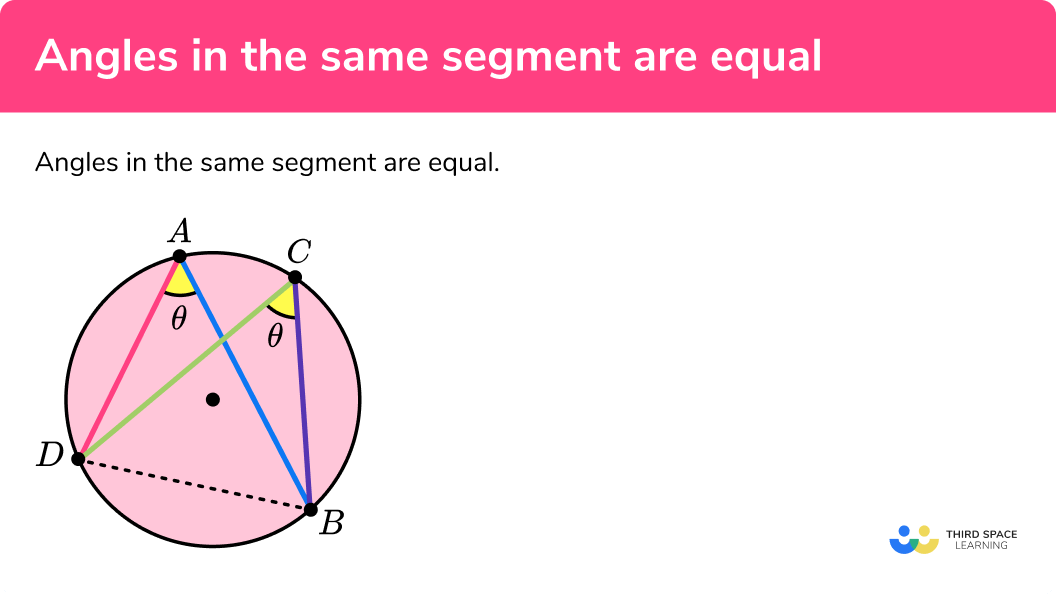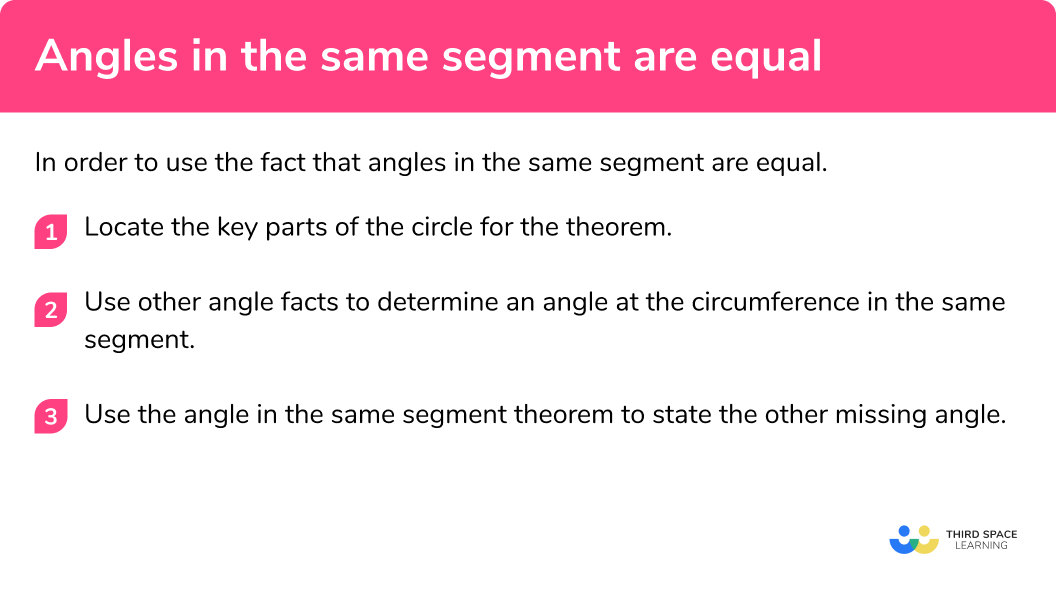GCSE Maths Geometry and Measure Circle Theorems

Angles In The Same Segment

# Angles In The Same Segment Are Equal

Here we will learn about the circle theorem: angles in the same segment, including its application, proof, and using it to solve more difficult problems.

There are also circle theorem worksheets based on Edexcel, AQA and OCR exam questions, along with further guidance on where to go next if you’re still stuck.

## What is angles in the same segment are equal?

Angles in the same segment are equal

In the diagram above, BD is a chord that divides the circle into the major and minor segments. The two points A and C on the circumference are joined to two other points on the circumference B and D . The angle DAB is the same as angle DCB . This is the same for any point that is placed on the major arc and so angles in the same segment are equal.

If the point is placed on the minor arc in the other segment, it would be a different angle, but all angles on the minor arc would be the same. Here, x=180-θ for any value of θ.

### What is angles in the same segment are equal?### Key parts of a circle needed for this theorem

Below is a diagram showing the key parts of a circle for this theorem:

• A chord is a straight line that meets the circumference in two places. The longest chord in a circle is the diameter.
• The major segment is the larger segment of a circle when it is enclosed by a chord and the major arc.
• The minor segment is the smaller part of a circle when it is cut by a chord and the minor arc.
• An arc is a part of the circumference. The major arc is longer than the minor arc.

Subtended angles

An angle within a circle is created by two chords meeting at a point on the circumference of a circle. The diagrams below show the angle subtended by arc AC from point B for two different circles.

### Proving that angles in the same segment are equal

To be able to prove the theorem, you need to know the following circle theorems:

• The angle at the centre is twice the angle at the circumference

1. We start by labelling the two angles at the circumference a and b

2Plot the centre of a circle and draw two radii from the centre to the circumference (here these are dashed lines). Label the angle between the two radii c .

3Let us inspect the angles a and c .

4We know that the angle at the centre is twice the angle at the circumference. This means that if the angle at the centre is equal to 2x, the angle at the circumference (which was our angle a)is now equal to half of 2x, or just x.

5Let us now inspect angles b and c .

6Again, we know that the angle at the centre is twice the angle at the circumference and so if we say that the angle c is equal to 2x, the angle b is equal to x.

7We now have the current situation where a and b are equal to x and the angle c is equal to 2x .

8This means that the two angles at the circumference are the same size and therefore we can state angles in the same segment are equal.

## How to use the angle in the same segment theorem

In order to use the fact that angles in the same segment are equal:

1. Locate the key parts of the circle for the theorem
2. Use other angle facts to determine an angle at the circumference in the same segment
3. Use the angle in the same segment theorem to state the other missing angle

### How to use the angle in the same segment theoremTangent of a circle is one of 7 circle theorems you will need to know. You may find it helpful to start with our main circle theorems page and then look in detail at the rest.

## Angles in the same segment examples

### Example 1: standard diagram

Below is a circle with centre O . AC and BD are chords. Calculate the size of angle CAD .

1. Locate the key parts of the circle for the theorem.

Here we have:

• The angle CBD = 47°
• AC and BD are chords
• The angle CAD = θ

2Use other angle facts to determine an angle at the circumference in the same segment.

We already know that DBC = 47° so we do not need to use any other angle fact to determine this angle for this example.

3Use the angle in the same segment theorem to state the other missing angle.

The angle CAD is in the same segment as the angle CBD and so we can state the angle of CAD

### Example 2: angles on a straight line

A, B, C, and D are points on a circle with centre O . Calculate the size of the angle CBD .

Locate the key parts of the circle for the theorem.

Use other angle facts to determine an angle at the circumference in the same segment.

Use the angle in the same segment theorem to state the other missing angle.

### Example 3: angles in a triangle

The circle with centre O has four points on the circumference. The two chords AC and BD intersect at the point E . Calculate the size of angle ABE .

Locate the key parts of the circle for the theorem.

Use other angle facts to determine an angle at the circumference in the same segment.

Use the angle in the same segment theorem to state the other missing angle.

### Example 4: angle at the centre

A, B, C, and D are points on the circumference of the circle with centre O . BOD is a straight line. Calculate the size of angle ABD .

Locate the key parts of the circle for the theorem.

Use other angle facts to determine an angle at the circumference in the same segment.

Use the angle in the same segment theorem to state the other missing angle.

ABCD are vertices of a cyclic quadrilateral. The circle has centre O . The chords AC and BD intersect at the point E . Calculate the size of the angle ABE .

Locate the key parts of the circle for the theorem.

Use other angle facts to determine an angle at the circumference in the same segment.

Use the angle in the same segment theorem to state the other missing angle.

### Example 6: complex diagram

A, B, C, D, and E are points on a circle with centre O . The lines AB and CD are parallel. Calculate the value of angle BDC .

Locate the key parts of the circle for the theorem.

Use other angle facts to determine an angle at the circumference in the same segment.

Use the angle in the same segment theorem to state the other missing angle.

### Common misconceptions

• Angle is double or half the original

The other angle in the same segment at the circumference is doubled or halved instead of it being the same.

• Angles add up to 90° or 180°

The two angles that are in the same segment at the circumference total 90° (or 180° if one angle is obtuse).

### Practice angles in the same segment questions

1. A circle with centre O has four points on the circumference, A, B, C, and D . Angle CAD = 11^{\circ} . Calculate the size of the angle CBD .10^{\circ}79^{\circ}22^{\circ}11^{\circ}CBD = CAD = 11^{\circ} (angles in the same segment are equal)

2. A, B, C, and D are points on the circle with centre O . The chords BD and AC intersect at the point E . Calculate the size of angle CBE .49^{\circ}47^{\circ}43^{\circ}50^{\circ}• BEC = 84^{\circ} (angles on a straight line)
• BCE = 47^{\circ} (angles in the same segment are equal)
• CBE = 180 – (84+47) = 49^{\circ} (angles in a triangle)

3. A, B, C, and D are points on the circle with centre O . BD and AC intersect at the point E . BD is not the diameter of the circle. Calculate the size of angle ABE .38^{\circ}51^{\circ}52^{\circ}44.5^{\circ}• CED = 89^{\circ} (angles on a straight line)
• DCE = 180 – (91+38) = 51^{\circ} (angles in a triangle)
• ABE = 51^{\circ} (angles in the same segment are equal)

4. A circle with centre O has four points on the circumference, A, B, C, and D . The reflex angle AOD = 306^{\circ} . Calculate the size of angle ABD .54^{\circ}27^{\circ}108^{\circ}44^{\circ}• AOD = 360 – 306 = 54^{\circ} (angles at a point)
• ACD = 54 ÷ 2=27^{\circ} (angle at the centre is twice the angle at the circumference)
• ABD = ACD = 27^{\circ} (angles in the same segment are equal).

5. A circle with centre O has four points on the circumference, A, B, C, and D . AC and BD intersect at the point E . Angle BCD = 84^{\circ} . Calculate the size of angle ABE .84^{\circ}52^{\circ}56^{\circ}42^{\circ}• BAD = 180 – 84 = 96^{\circ} (opposite angles in a cyclic quadrilateral total 180^{\circ} )
• BAE = 96 – 52 = 44^{\circ}
• ABE = 180 – (80+44) = 56^{\circ} (angles in a triangle)

6. A circle with centre O has points A, B, C, D, and E on the circumference. Angle BAF = 58^{\circ} . Calculate the size of the angle BCD .122^{\circ}64^{\circ}174^{\circ}116^{\circ}• BCD = 180 – 58 = 122^{\circ} (angles in opposing segments total 180^{\circ} in a cyclic quadrilateral).

### Angles in the same segment GCSE questions

1. Use the information in the diagram below to calculate the size of angle ACB. Explain your answer.(4 marks)

ADB = 180 – (78 + 65) = 37^∘

(1)

Angles in a triangle total  180^∘

(1)

(1)

Angles in the same segment are equal

(1)

2. A, B, C, D are points on the circle with centre O. The chords AC and BD intersect at the point E. The tangent FG goes through the point B. Terry says that angle ACD = 11^∘. Is he correct?(5 marks)

ABE = 90 – 75 = 15^∘

(1)

The tangent meets the radius at a right angle

(1)

θ = ACD = ABD = 15^∘

(1)

Angles in the same segment are equal

(1)

No, Terry is wrong

(1)

3 (a). The circle with centre O has 4 points on the circumference, A, B, C, and D. Calculate the size of angle ADB.(b). Hence state the size of angle AED in the diagram below.(6 marks)

(a) BAC = 68\div 2 = 34^∘

(1)

Angle at the centre is twice the angle at the circumference

(1)

180 – (34 + 42) = 104^∘

(1)

Angles in a triangle total 180^∘

(1)

(b) AED = 42^∘

(1)

Angles in the same segment are equal

(1)

## Learning checklist

You have now learned how to:

• Apply and prove the standard circle theorems concerning angles, radii, tangents and chords, and use them to prove related results

## Still stuck?

Prepare your KS4 students for maths GCSEs success with Third Space Learning. Weekly online one to one GCSE maths revision lessons delivered by expert maths tutors.

Find out more about our GCSE maths tuition programme.